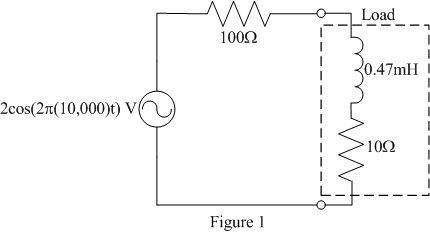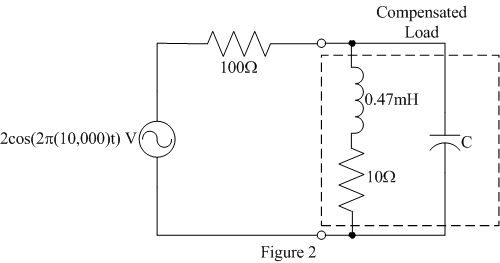EE 212 Lab
Lab 5: Power Factor Correction

Recall that average power absorbed by a circuit element is VrmsIrmscos(¸) where ¸ is the phase difference between the element's sinusoidal voltage v(t) and current i(t). To obtain the most efficient use of the current delivered to a load, it is desired that the load voltage and current be in phase (¸ = 0, pf = 1) such that average power is maximized. The purpose of this lab is to add compensation to an inductive load such that its equivalent power factor becomes close to 1.

Notes on Measurements:

• Experimental determination of the power factor typically requires the measurement of the phase difference between voltage and current. Two properties of the function generator and oscilloscope make this measurement difficult.
• The reference (ground) leads on the oscilloscope and function generator are internally tied to ground making it imperative that they all be connected to the same point in the circuit.
• The difference between channels 1 and 2 (CH1-CH2) can only be displayed by itself on the oscilloscope.
• Carefully consider where to take measurements such that subject to the above constraints the information of interest can be determined.

Laboratory Procedure: